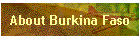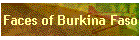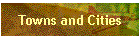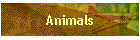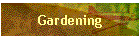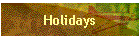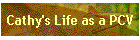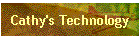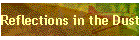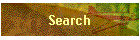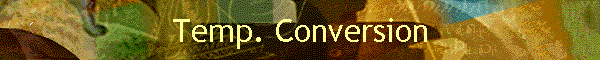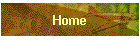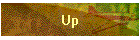A quick lesson on how to convert between temperatures in degrees Fahrenheit and degrees Celsius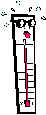Converting between units of measure or scales is a nice use of algebraic formulas. When a formula represents one quantity (such as temperature in degrees Fahrenheit) in terms of another quantity (such as temperature in degrees Celsius), it shows a functional relationship. Thinking of algebra in terms of functions like this helps algebra make sense.

If you don't want to compute with fractions, use a calculator or make a computer spreadsheet. Better yet, use the mental technology you always have with you (in your head), and learn the mental shortcuts below.

 To convert from Celsius to Fahrenheit:Multiply the temperature in degrees Celsius by 9/5, then add 32 (degrees) to find the temperature in degrees Fahrenheit.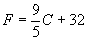*A mental shortcut for a rough estimate: Double the temperature given in Celsius and add 30 to the result to find the approximate temperature in Fahrenheit. To convert from Fahrenheit to Celsius:Subtract 32 (degrees) from the temperature in degrees Fahrenheit, then multiply by 5/9 to find the temperature in degrees Celsius.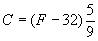*A mental shortcut for a rough estimate: Subtract 30 from the temperature given in Fahrenheit and take half of the result to find the approximate temperature in Celsius. Can you explain why these shortcuts work? If you want to remember these formulas or the shortcuts, think of the temperatures for freezing and boiling  water in Celsius (0 and 100) and Fahrenheit (32 and 212) as a reminder of whether to add or subtract and whether to multiply by 5/9 or 9/5.  And don't forget that the mental shortcuts only give a rough estimate. Some sample conversions (Can you justify the last three?):40 � C = ? � F        >>>>>       F = 9/5 x 40 + 32 = 72 + 32 = 104 � F 35 � C = 95 � F 30 � C = 86 � F 25 � C = 77 � F Try the following conversions: 20 � C = ? � F ? � C = 100 � F 38 � C = ? � F ? � C = 32 � F